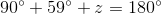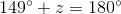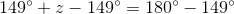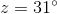# SSAT Upper Level Math : How to find an angle in a right triangle

## Example Questions

### Example Question #1 : How To Find An Angle In A Right Triangle

One angle of a right triangle has measure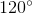. Give the measures of the other two angles.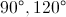This triangle cannot exist.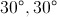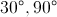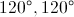This triangle cannot exist.

Explanation:

A right triangle must have one right angle and two acute angles; this means that no angle of a right triangle can be obtuse. But since, it is obtuse. This makes it impossible for a right triangle to have aangle.

### Example Question #51 : Right Triangles

One angle of a right triangle has measure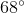. Give the measures of the other two angles.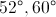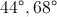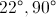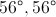This triangle cannot exist.Explanation:

One of the angles of a right triangle is by definition a right, or, angle, so this is the measure of one of the missing angles. Since the measures of the angles of a triangle total, if we let the measure of the third angle be, then: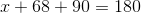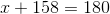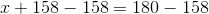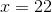The other two angles measure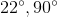.

### Example Question #3 : How To Find An Angle In A Right Triangle

Find the degree measure ofin the right triangle below.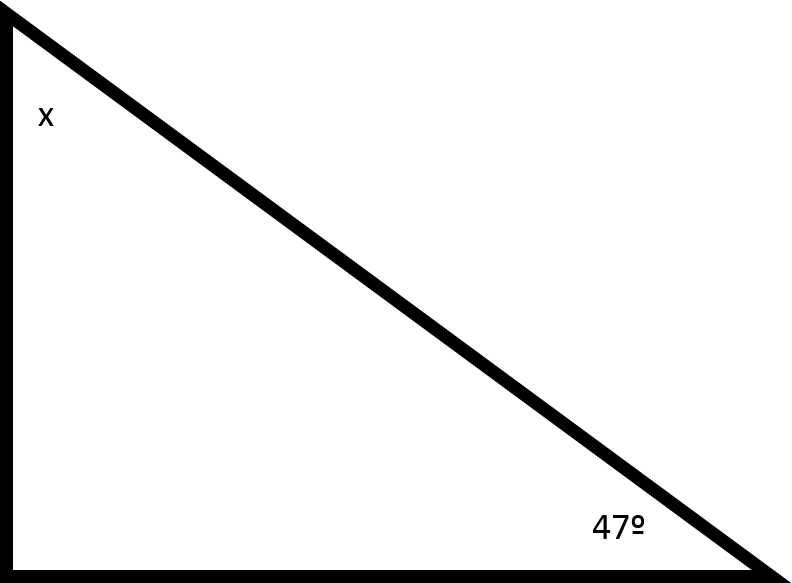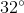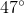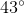Explanation:

The total number of degrees in a triangle is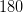.

Whileis provided as the measure of one of the angles in the diagram, you are also told that the triangle is a right triangle, meaning that it must contain aangle as well. To find the value of, subtract the other two degree measures from.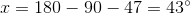### Example Question #4 : How To Find An Angle In A Right Triangle

Find the angle value of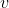.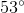Explanation:

All the angles in a triangle must add up to 180 degrees.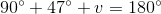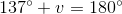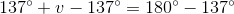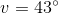### Example Question #5 : How To Find An Angle In A Right Triangle

Find the angle value of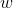.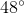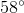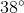Explanation:

All the angles in a triangle adds up to.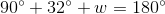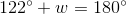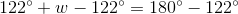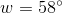### Example Question #6 : How To Find An Angle In A Right Triangle

Find the angle value of.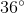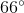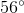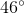Explanation:

All the angles in a triangle add up todegrees.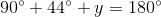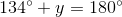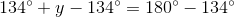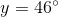### Example Question #7 : How To Find An Angle In A Right Triangle

Find the angle measure of.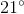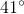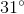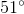Explanation:

All the angles in a triangle add up to.